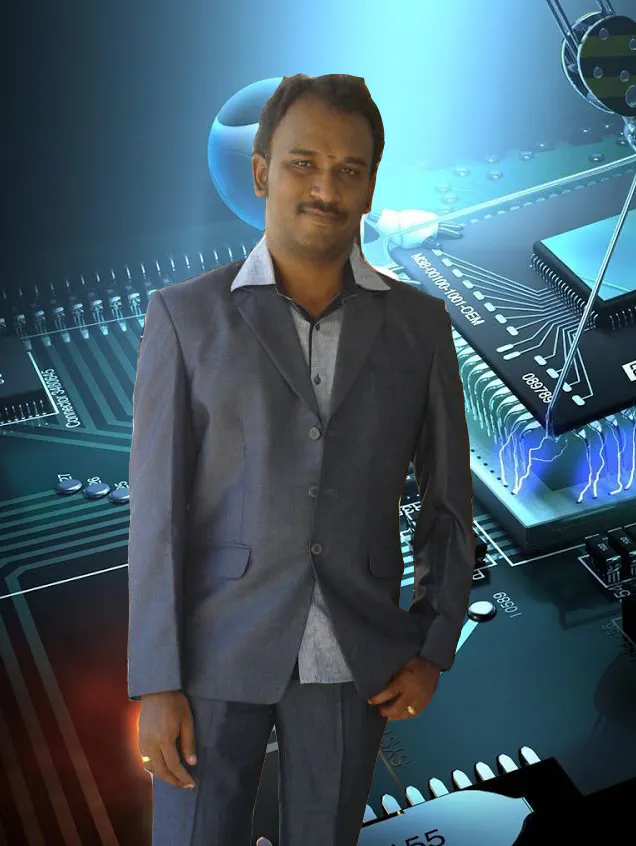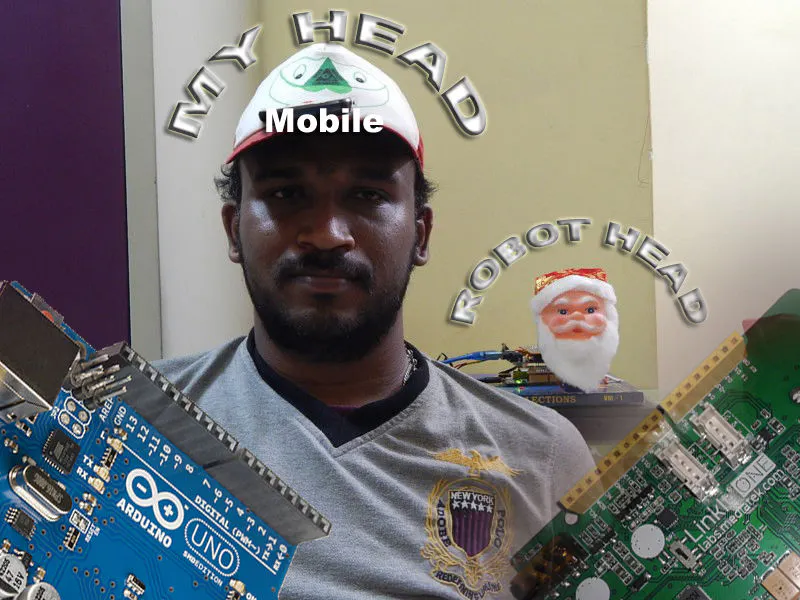My Dream humanoid robot where what we done in a room is done by a robot in the remote. First step is start from head.## Things used in this project

### Hardware componentsArduino UNO & Genuino UNO
×1
 servo motor
×3Android device
×1
 Regulated Power supply board
×1

### Software apps and online services

 SensoDuino

### Hand tools and fabrication machinesSoldering iron (generic)

## Code

### Servoposition.ino

Arduino
```#include <Servo.h>
Servo leftright;
Servo updown;
Servo shake;

void setup() {
Serial.begin(9600);

leftright.attach(3);
updown.attach(5);
shake.attach(6);
leftright.write(90);
updown.write(90);
shake.write(90);
}

void loop() {

}
```

Arduino
```#include <LBT.h>
#include <LBTServer.h>

String inputString = "";
String outputString = "";

int firstpoint=0;
int secondpoint=0;

void setup() {
if(!LBTServer.begin((uint8_t*)"Sivam_LIO"))
{
return;
}
// Serial.begin(9600);
Serial1.begin(9600);
}
void loop() {
uint8_t buf;
if(LBTServer.connected())
{
while(true)
{
break;
inputString="";
for(int j=0;j<32;j++)
{
char inChar = char(buf[j]);
// add it to the inputString:
inputString += inChar;
}
// Serial.println(inputString);
Serial1.println(inputString);
// firstpoint=inputString.indexOf('>');
// Serial.println(inputString);
// Serial.println(firstpoint);
// if (firstpoint>0)
// {
//   secondpoint=inputString.indexOf('>',firstpoint+1);
//   if (secondpoint>0)
//   {
//     outputString=inputString.substring(firstpoint,secondpoint);
//     inputString=inputString.substring(secondpoint);
//     Serial.println(outputString);
//   }
// }

}
delay(100);
}
else
{
LBTServer.accept(5);
}
}
```

Arduino
```#include <Servo.h>
Servo leftright;
Servo updown;
Servo shake;

String inputString = "";
boolean stringComplete = false;

String teststr2;
String sensorid;
String sensorrecid;
String sensorval1;
String sensorval2;
String sensorval3;

int S1;
int S2;
int S3;

int startchr=0;
int endchr=0;

int rot1;
int rot2;
int rot3;

void setup() {
Serial.begin(9600);
//  inputString.reserve(200);

leftright.attach(3);
updown.attach(5);
shake.attach(6);
}

void loop() {
}

void serialEvent() {
while (Serial.available()) {
if (inChar != '\n') {
inputString += inChar;
}
}

startchr = inputString.indexOf('>');
if (startchr>=0)
{
endchr = inputString.indexOf('>',startchr+1);
if (endchr>0)
{
teststr2=inputString.substring(startchr,endchr);
inputString=inputString.substring(endchr);
startchr = teststr2.indexOf(',');
sensorid = teststr2.substring(1,startchr);

endchr = teststr2.indexOf(',',startchr+1);
sensorrecid=teststr2.substring(startchr+1,endchr);
startchr=endchr;

endchr = teststr2.indexOf(',',startchr+1);
sensorval1=teststr2.substring(startchr+1,endchr);
startchr=endchr;

endchr = teststr2.indexOf(',',startchr+1);
sensorval2=teststr2.substring(startchr+1,endchr);
startchr=endchr;

sensorval3=teststr2.substring(startchr+1);

S1=sensorval1.toInt();
S2=sensorval2.toInt();
S3=sensorval3.toInt();

rot1=map(S1, 90, 270, 180, 0);
if (rot1<0)
rot1=0;
else if (rot1>180)
rot1=180;

rot2=map(S2, -90, 90, 0, 180);
if (rot2<0)
rot2=0;
else if (rot2>180)
rot2=180;

rot3=map(S3, 90, -90, 0, 180);
if (rot3<0)
rot3=0;
else if (rot3>180)
rot3=180;

if (rot1!=0 & rot1!=180)
{
leftright.write(rot1);
}
if (rot1!=0 & rot1!=180)
{
updown.write(rot2);
}
if (rot1!=0 & rot1!=180)
{
shake.write(rot3);
}
delay(15);
//      Serial.print("Sensor-");
//      Serial.println(sensorid);
//      Serial.print("Sensor Val1-");
//      Serial.println(rot1);
//      Serial.print("Sensor Val2-");
//      Serial.println(rot2);
//      Serial.print("Sensor Val3-");
//      Serial.println(rot3);
}
}
}
```

## Credits

### jegatheesan

17 projects • 46 followers
Simply A Mechatronics Lover.